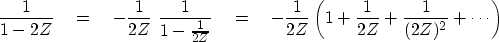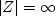Next: Inverse filters Up: INSTABILITY Previous: INSTABILITY

## Anticausality

Luckily, unstable filters can be made stable as follows:(43)
Equation (43) is a series expansion in 1/Z--in other words, a series about infinity. It converges fromall the way in to a circle of radius |Z|=1/2. This means that the inverse converges on the unit circle where it must, if the coefficients are to be bounded. In terms of filters, it means that the inverse filter must be one of those filters that responds to future inputs. Hence, although it is not physically realizable, it may be used in computer simulation.

Examining equations (42) and (43), we see that the filter 1/(1-2Z) can be expanded into powers of Z in (at least) two different ways. Which one is correct? The theory of complex variables shows that, given a particular numerical value of Z, only one of the sums (42) or (43) will be finite. We must use the finite one, and since we are interested in Fourier series, we want the numerical value |Z|=1 for which the first series diverges and the second converges. Thus the only acceptable filter is anticausal.

The spectra plotted in Figure 11 apply to the anticausal expansion. Obviously the causal expansion, which is unbounded, has an infinite spectrum.

We saw that a polynomial B(Z) of degree N may be factored into N subsystems, and that the ordering of subsystems is unimportant. Suppose we have factored B(Z) and found that some of its roots lie outside the unit circle and some lie inside. We first invert the outside roots with equation (41) and then invert the inside roots with equation (43). If there are any roots exactly on the unit circle, then we have a special case in which we can try either inverse, but neither may give a satisfactory result in practice. Implied zero division is nature's way of telling us that what we are trying to do cannot be done that way (if at all).Next: Inverse filters Up: INSTABILITY Previous: INSTABILITY
Stanford Exploration Project
10/21/1998